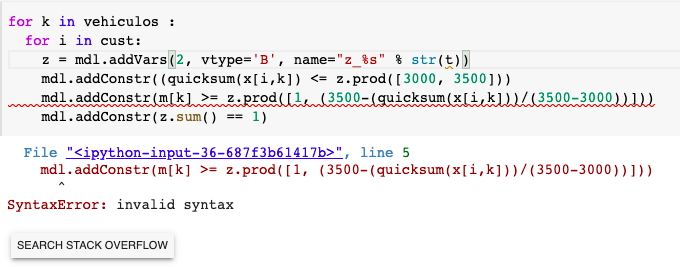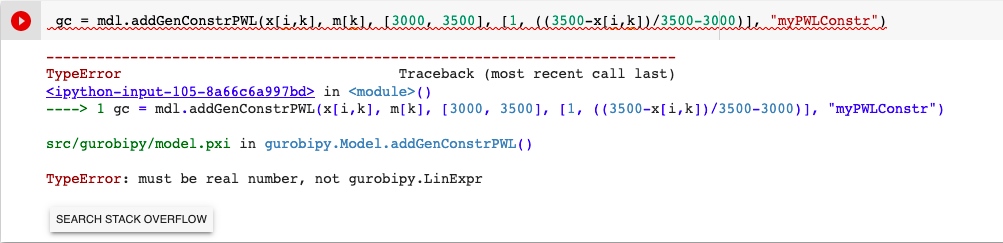•Gurobi Staff

Hi Putri,

It is not clear to me what exactly you are trying to achieve. Could you please elaborate more on what exactly you are trying to model/implement in Gurobi?

Best regards,
Jaromił

•Hi, sorry for the unclear question. I want to ask how to model the membership value of fuzzy logic in Gurobi. In my case, I want to assign the membership value (mv) based on the current quantity (x) with the parameter (a) = desired capacity and (b) = maximum capacity.

if the current quantity in vehicle <=a, then mv = 1

if the current quantity in vehicle < b, then mv = 1/ ((b-x)/(b-a))

then the mv will be included in the obj function:

mdl.setObjective(quicksum(y[i,j,k]*traveltime[i,j]*mv[k] for i,j,k in q))

note: I have 4 vehicles; i,j represent the nodes and k represent the vehicle

•Gurobi Staff

Hi Putri,

Thank your for the clarification. What you are trying to model is the piecewise-linear function

\begin{align*} z = \begin{cases} \frac{r-a}{b-a}&, a \leq r \leq b \\ 1&, b \leq r \leq c\\ 1 - \frac{r-a}{b-a} &, c \leq r \leq d\end{cases} \end{align*}

This can be implemented using the addGenConstrPWL method. I would recommend having a look at our documentation on piecewise-linear objectives where we explain modeling piecewise-linear functions.

In general, you will have to add an auxiliary variable $$z$$ and compute values at the border points of the segments of the above piecewise-linear function and feed this information to the addGenConstrPWL method.

Best regards,
Jaromił

•It works well until I modified the code for my research and I got this error:I've been using 'mdl' for the whole code, and this is the only error. Could you please help me?

•Gurobi Staff

Hi Putri,

Python's error messages can be a bit cryptic here, and often point to the code after the issue, not the issue itself. In this case it looks like you just have a mismatched bracket at this line which causes the syntax error:

mdl.addConstr((quicksum(x[i,k]) <= z.prod([3000, 3500]))#             ^ this second bracket isn't needed

Best regards,
Simon

•Hi Simon,

It works! Thank you.

•Gurobi Staff

Hi Putri,

Please note that with the constraints from the stackexchange post, you are modeling nothing different than a piecewise-linear function, which can be modeled automatically using Gurobi's addGenConstrPWL method. In case that you are going to model this logic more often, I would recommend having a closer look at piecewise-linear functions and their modeling in Gurobi to make your model construction easier and less error prone.

Best regards,
Jaromił

•Hi Jaromil,

I think so. I revised the constraints into the following:but I encountered a problem to define this. The x[i,k] is the current capacity and m[k] will be the returned value according to the position of x[i,k] .

•Gurobi Staff

Hi Putri,

Your input does not work because you have to provide real values for x points and y points of the PWL function, i.e., no optimization variables are allowed as input for the 3rd and 4th arguments, see addGenConstrPWL documentation.

mdl.addGenConstrPWL(r, x, [20,30,40,40,50], [(20-20)/(30-20),(30-20)/(30-20),1,1-(40-20)/(30-20),1-(40-20)/(30-20)])

Note that I added two values for the point $$r=40$$, because there is a jump at $$r=40$$ from $$1$$ to $$1-\frac{40-20}{30-20} = -1$$. You can find all details in our documentation on piecewise-linear objectives.

Best regards,
Jaromił

•Hi Jaromil,

Thank you for your great help! It's much simpler and it works!